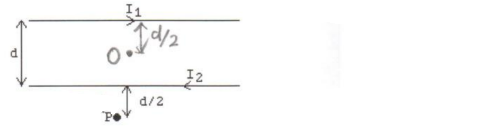🤓 Based on our data, we think this question is relevant for Professor Lumata's class at UTD.

# Solution: In the figure, the two long straight wires are separated by a distance of d = 0.40 m. The currents are I1 = 1.0 A to the right in the upper wire and I 2 = 8.0 A to the left in the lower wire. Find the magnitude and direction of the magnetic field at the center point O, and also point P, a distance d/2 = 0.20 m below the lower wire? (NOTE: μ0 = 4π x 10-7 T•m/A.)

###### Problem

In the figure, the two long straight wires are separated by a distance of d = 0.40 m. The currents are I1 = 1.0 A to the right in the upper wire and I 2 = 8.0 A to the left in the lower wire. Find the magnitude and direction of the magnetic field at the center point O, and also point P, a distance d/2 = 0.20 m below the lower wire? (NOTE: μ0 = 4π x 10-7 T•m/A.)